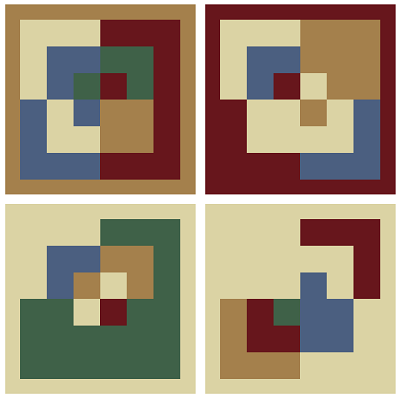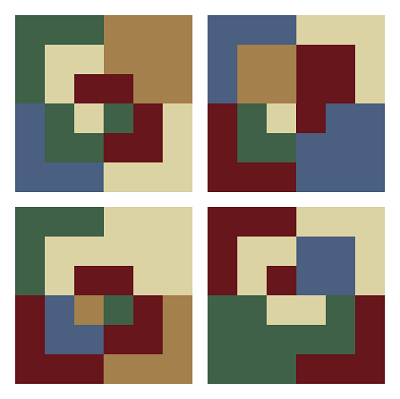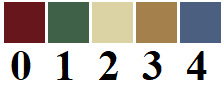# Algorithmic Art

 Behind the scenes of Algorithm + You = Art How do I use your input to create art? That's a good question with a complicated answer. It is impossible to fully answer that question since each script uses the input differently. However, here is an example of how I use your input in one of the scripts. Shown below is the important part of the script 454 Quartered Corner Concentric Squares (The code is simplified a little bit to make it easier to understand). ``````for row in range(0, numRows): for col in range(0, numCols): x = col*cellSize+rad+border y = row*cellSize+rad+border for i in range(1, 5): for j in range(0, k): size=(k-j)*r/k) pick=random.choice(palette) turt.color(pick) drawQuad(turt,x,y,size,i)`````` This script generated the following image using randomness. In other words, if I ran it again, the image would almost certainly be completely different.It uses random numbers to determine what color each quarter of the squares will be. This is done on the line that reads ` pick=random.choice(palette)` which is saying to randomly choose a color from the color palette. Script 503 Quartered Corner Concentric Squares, a portion of which is shown below, is almost identical to script 454. This is the one that is part of Algorithms + You = Art. ``````for row in range(0, numRows): for col in range(0, numCols): x = col*cellSize+rad+border y = row*cellSize+rad+border for i in range(1, 5): for j in range(0, k): size=(k-j)*r/k) pick=palette[in.nextBits(b)%n] turt.color(pick) drawQuad(turt,x,y,size,i)`````` Notice that the only line of code that is different is ` pick=palette[in.nextBits(b)%n]` which we will explain in more detail below. The important thing right now is that instead of using randomness to determine what color each quarter square will be, it relies on user input (i.e. words and/or phrases). Running this script with input "Hello World!" and color palette EdwardHopper (5) produces the following image.Every time I run the script with this input, I will get exactly the same output because instead of using randomness, I use the encoding of the input as described below. As mentioned, the example above was produced using input "Hello World!", which when represented in a format called UTF-8 is 01001000 01100101 01101100 01101100 01101111 00100000 01010111 01101111 01110010 01101100 01100100 00100001. (In case you do not already know this, computers store everything in terms of 0s and 1s.) Instead of using random numbers like script 454 does, this script uses the bits in the UTF-8 representation to determine colors. In this case, I chose a color palette that has 5 colors. To choose a color, I need to pick a number between 0 and 4. (Computer scientists like to start counting from 0 instead of 1 for a very important reason that we won't go into here.) The number 4 takes 3 bits to represent in binary (it is 100). Therefore, I use 3 bits at a time to determine what color to use. The code `in.nextBits(b)` is asking for the next 3 bits from the input (because `b=3` in this case). Notice that with 3 bits, the numbers 5 (101), 6 (110), and 7 (111) are possible in addition to 0 (000), 1 (001), 2 (010), 3 (011), and 4 (100). Because I only have 5 colors (numbered 0 through 4), 5, 6, and 7 are a problem. I take the easy way out—I just convert 5, 6, and 7 to 0, 1, and 2 using something called modular arithmetic. In the code this is represented by `%n`, where `n=5` in this case. So the code `in.nextBits(b)%n` gives a number between 0 and 4 based on the next 3 bits from the input. The square brackets indicate array indexing, which is just asking for the item with the given number from the list. For instance, `palette` is the first item, `palette` is the second item, etc. In this example, the first color is chosen based on the first 3 bits, 010. This represents 2. I chose the color palette displayed below, so color 2 is the light tan color.Now for the slightly harder part. Look closely at the second image above. The first part that is draw is the lower-left square (row 0 and column 0). Of that square, the upper right corner is drawn first (the first quadrant, a term that should be familiar if you ever took a pre-calculus course), and it is drawn from outside to inside (large square, medium square, small square). So the first three colors are light tan, light tan, and dark red. Do you see it? As we already determined, light tan should be the first color. Notice that the next bits of input are 010 and 000, which correspond to 2 and 0, which yield light tan and dark red! So there is method in my madness! If you think about it for a minute, you will realize that colors 0, 1, and 2 will come up more often than colors 3 and 4. There is not an easy way around this problem, and it does not seem to make the art look any worse, so I just accept this limitation rather than implementing a more complicated scheme that will ensure all colors occur with the same frequencies (Not only would it be more complicated, it would "eat up" more bits, and I don't like that). Of course, if you use a color palette with 4 or 8 colors, this won't be a problem! At least for this particular script. Each script has a certain number of colors that work best. But don't overthink it. Of course, there is a lot more to the various scripts than what I describe here. But my main goal was to give you a glimpse of how your input is used to create art, and hopefully I have done that! O.K., now it's time to Make art!